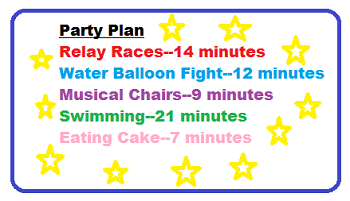# Rounding Numbers: Lesson for Kids

Instructor: Bethany Calderwood

Bethany has taught special education in grades PK-5 and has a master's degree in special education.

Rounding numbers is a way to make mental math easier. In this lesson, you will learn to simplify numbers by rounding them to the tens place and to use these rounded numbers to make estimates.

## What Is Rounding?

When we talk about rounding, we're talking about simplifying a number. Rounding involves making a number simpler by changing it to its closest value that's easier to work with, which is particularly helpful when you're dong mental math. For instance, if you need to find the answer to 71 x 5, you might round 71 to 70, making it easier to do the math in your head and find an answer of 350.

When you round numbers before solving a problem, such as addition or subtraction, you are estimating. Estimation is an approximate calculation. The results of estimating may be less accurate, but it's useful in situations where a precise calculation isn't necessary.

## Rules of Rounding

You can choose any place value to round a number to, depending on your needs. In this lesson, we will learn the rules for rounding to the tens place, which means we want to round numbers to multiples of ten: 10, 20, 30, 40, etc. To round to the tens place, there are two simple rules to remember:

• If there's a 4 or lower in the ones place, you should round down. For instance, you would round the number 64 down to 60.
• If there's a 5 or higher in the ones place, you should round up. For instance, you would round 85 up to 90.

These rules can be applied to different place values when rounding to different place values. For instance, if you're rounding to the hundreds, you would apply the four-or-lower and five-or-higher rules the tens place. Take the number 487. Since there's an 8 in the tens place, you would round up to the nearest hundred: 500.

## Rounding Exercise: Planning a Party

Ramon is planning a party. He has a list of activities and their exact times, and he wants to estimate how much of the party will be taken up by these activities. Ramon will use rounding to simplify the numbers before adding them together in his head. Let's start by rounding the minutes to the tens for each activity:To unlock this lesson you must be a Study.com Member.

### Register to view this lesson

Are you a student or a teacher?

#### See for yourself why 30 million people use Study.com

##### Become a Study.com member and start learning now.
Back
What teachers are saying about Study.com

### Earning College Credit

Did you know… We have over 200 college courses that prepare you to earn credit by exam that is accepted by over 1,500 colleges and universities. You can test out of the first two years of college and save thousands off your degree. Anyone can earn credit-by-exam regardless of age or education level.